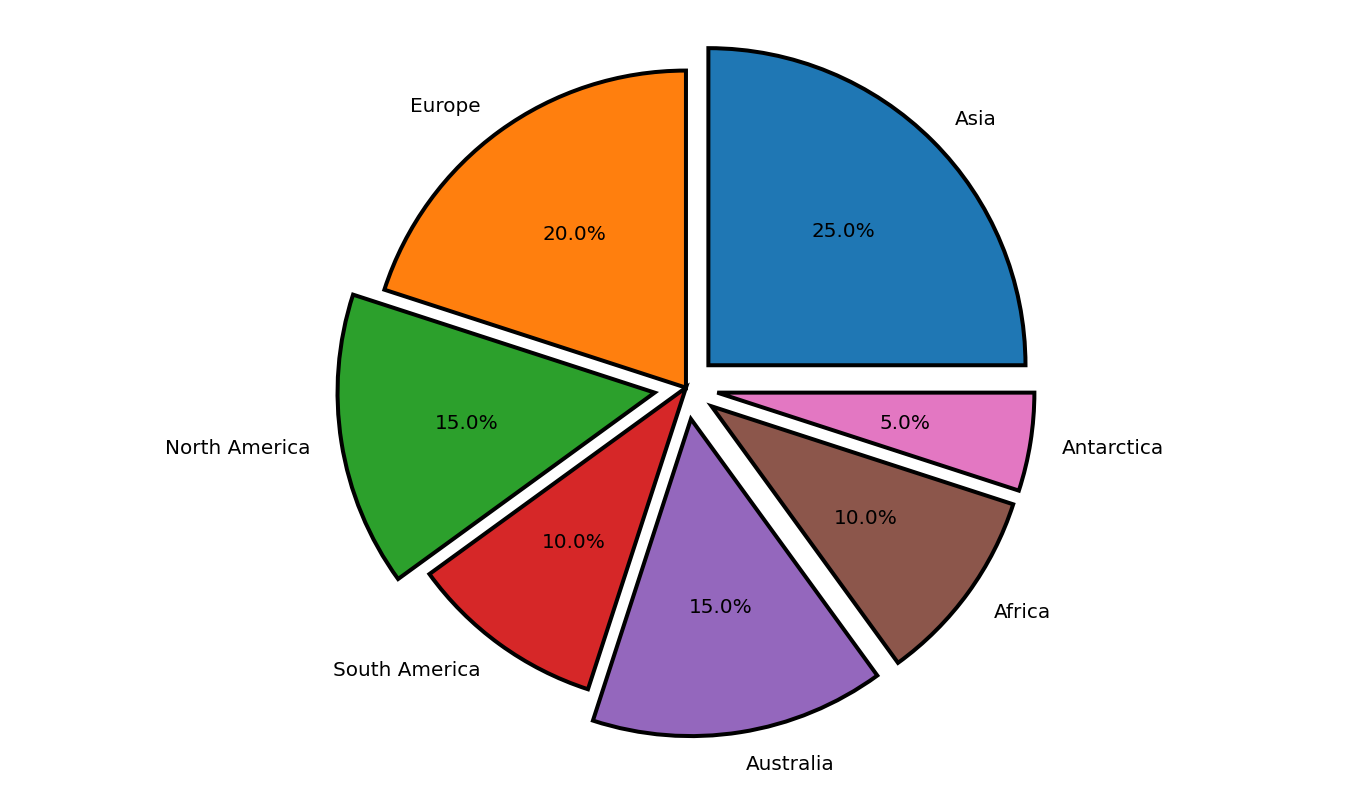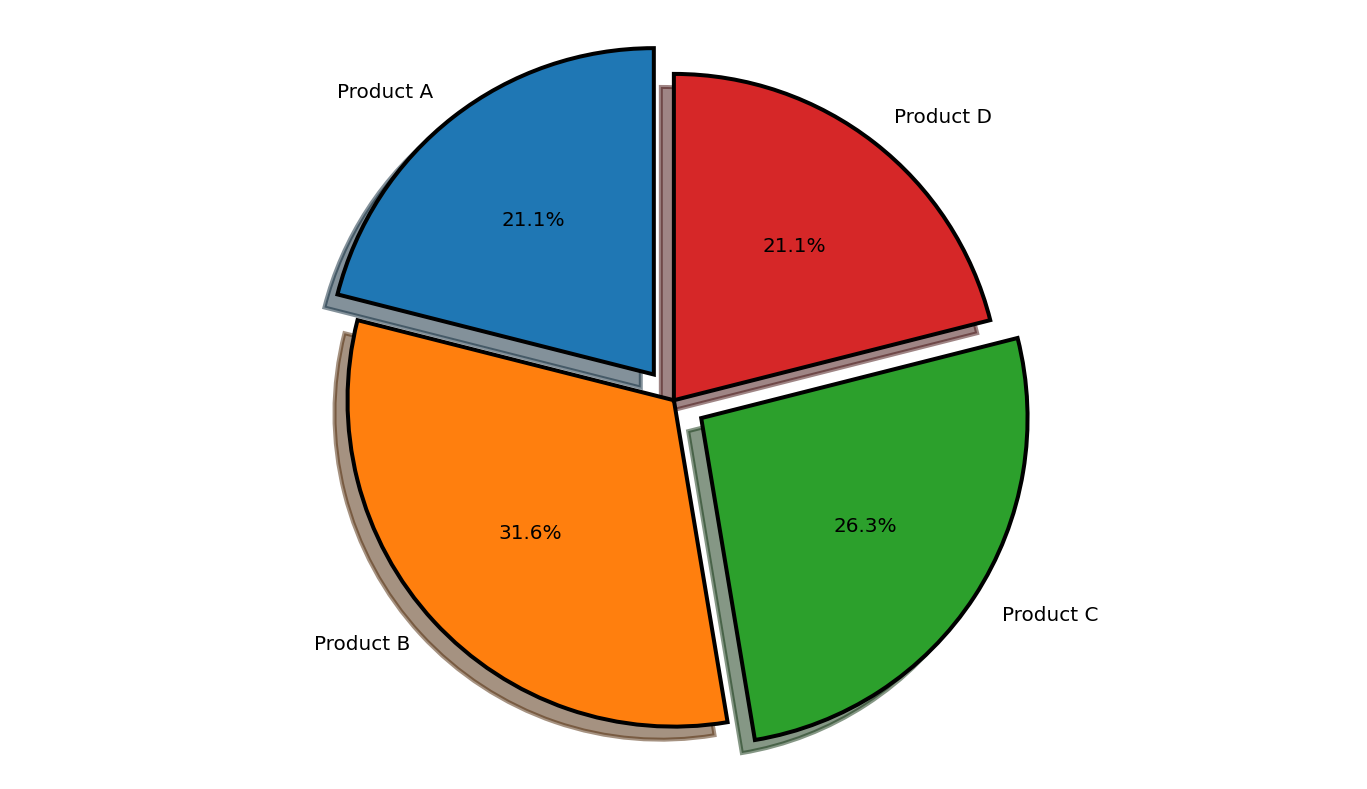# Radially displace pie chart wedge in Matplotlib

• Last Updated : 17 Dec, 2020

Pie charts are statistical graphs divided into slices that represent different data values and sum up to 100%. Python is one of the most popularly used programming languages for data visualization. Python has multiple data visualization libraries and Matplotlib is one of them. Matplotlib is widely used because of its simplicity and ease of implementation.  The matplotlib library supports pie chart creation. The explode feature allows separating the slices of the piechart. Users may explicitly specify the portion they wish to explode. The value specified in the explode tuple decides the distance to which the slice is exploded. More the value greater the distance of the slice from the center of the pie.

Example 1:

In this example, the matplotlib.pyplot module is imported. The continents and area arrays are initialized. The explode tuple is defined. The indexes of the slices which must be split are given a value greater than 0. The slice is moved at a distance specified in the tuple from the center of the circle. The pie chart is plotted for the specified data. The autopct attribute is used to specify the distance of the text in the slice from the center of the circle. The startangle is used to specify the orientation of the chart. The default value of startangle is 0 which means the plotting starts at positive X axis. The edge color is set to black and linewidth is set to 2. The anti-aliasing property is set to true to prevent the aliasing effect on the image.

Below is the Implementation:

## Python3

 `import` `matplotlib.pyplot as plt`` ` `# the slices are ordered and ``# plotted counter-clockwise:``continents ``=` `[``'Asia'``, ``'Europe'``, ``'North America'``,``              ``'South America'``,``'Australia'``,``              ``'Africa'``,``'Antarctica'``]`` ` `area ``=` `[``25``, ``20``, ``15``, ``10``,``15``,``10``,``5``]``explode ``=` `(``0.1``, ``0``, ``0.1``, ``0``,``0.1``,``0.1``,``0.1``) `` ` `plt.pie(area, explode ``=` `explode, labels ``=` `continents,``        ``autopct ``=` `'%1.1f%%'``,startangle ``=` `0``,``        ``wedgeprops ``=` `{``"edgecolor"` `: ``"black"``,``                    ``'linewidth'` `: ``2``,``                    ``'antialiased'``: ``True``})`` ` `# Equal aspect ratio ensures ``# that pie is drawn as a circle.``plt.axis(``'equal'``) `` ` `plt.show()`

Output:Example 2:

In this example, the matplotlib.pyplot module is imported. The sales and profit arrays are initialized. The explode tuple is defined. The indexes of the slices which must be split are given a value greater than 0. The slice is moved at a distance specified in the tuple from the center of the circle. The pie chart is plotted for the specified data. The autopct attribute is used to specify the distance of the text in the slice from the center of the circle. The startangle is used to specify the orientation of the chart. The default value of startangle is 0 which means the plotting starts at the positive X axis. The edge color is set to black and linewidth is set to 2. The anti-aliasing property is set to true to prevent an aliasing effect on the image.

Below is the Implementation:

## Python3

 `import` `matplotlib.pyplot as plt`` ` `# the slices are ordered and plotted counter-clockwise:``sales ``=` `[``'Product A'``, ``'Product B'``, ``         ``'Product C'``, ``'Product D'``]`` ` `profit ``=` `[``20``, ``30``, ``25``, ``20``]``explode ``=` `(``0.1``, ``0``, ``0.1``, ``0``) `` ` `plt.pie(profit, explode ``=` `explode, labels ``=` `sales,``        ``autopct ``=` `'%1.1f%%'``,shadow ``=` `True``,``        ``startangle ``=` `90``,``        ``wedgeprops ``=` `{``"edgecolor"``:``"black"``,``                    ``'linewidth'``: ``2``,``                    ``'antialiased'``: ``True``})`` ` `# Equal aspect ratio ensures``# that pie is drawn as a circle.``plt.axis(``'equal'``)  `` ` `plt.show()`

Output:My Personal Notes arrow_drop_up